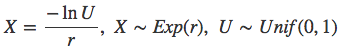# RunKit +#### Try any Node.js package right in your browser

This is a `playground` to test code. It runs a full `Node.js` environment and already has all of `npm`’s 1,000,000+ packages pre-installed, including `poisson-process` with all `npm` packages installed. Try it out:

var poissonProcess = require("poisson-process")

This service is provided by RunKit and is not affiliated with npm, Inc or the package authors.

# poisson-process.jsA JavaScript library to generate naturally varying time intervals. It improves realism and natural unpredictability in your games or animations like aliens walking by a window, or cars trying to drive over your character on a busy road. It removes bottlenecks in distributed systems by adding jitter that prevents thundering herd problem. It can also simulate the frequency of chat messages, page loads or arriving emails as well as queues, traffic and earthquakes. The underlying mathematical concept is called the Poisson process.In the animation above, the blue cars drive by in constant time intervals and the red ones in more natural, randomized intervals typical for the Poisson process.

## Installation

### Browsers

``````<script src="poisson-process.min.js"></script>
``````

### Node.js & CommonJS

First `\$ npm install poisson-process` and then:

``````var poissonProcess = require('poisson-process');
``````

### AMD & Require.js

``````define(['scripts/poisson-process'], function (poissonProcess) { ... });
``````

## Usage

It is simple; you specify an average call interval in milliseconds, a function to be called and then start the process.

``````> var p = poissonProcess.create(500, function message() {
console.log('A message arrived.')
})
> p.start()
``````

Now the `message` function will be called each 500 milliseconds in average. The delay from a previous call can vary from near 0 milliseconds to a time that is significantly longer than the given average, even though the both ends are increasingly unlikely.

The process is paused by:

``````> p.stop()
``````

If you desire just numbers, generate intervals by:

``````> poissonProcess.sample(500)
389.33242512
> poissonProcess.sample(500)
506.58621391
``````

## API

### poissonProcess.create(averageIntervalMs, triggerFunction)

The `create` constructor takes in two parameters. The `averageIntervalMs` is an integer and the average interval in milliseconds to call the `triggerFunction`. The `triggerFunction` takes no parameters and does not have to return anything.

``````var p = PoissonProcess.create(500, function message() {
console.log('A message arrived.')
})
``````

### p.start()

Start the process; begin to call the `triggerFunction`.

``````p.start()
``````

### p.stop()

Stop the process; do not anymore call the `triggerFunction`.

``````p.stop()
``````

### poissonProcess.sample(average)

The `sample` provides a raw access to the underlying generator for the call intervals. It returns a number; a sample from the exponential distribution with the rate `1 / average`.

``````> poissonProcess.sample(500)
323.02...
> poissonProcess.sample(500)
returns 941.33...
> poissonProcess.sample(500)
returns 609.86...
``````

## Theory

The poisson-process.js is based on the mathematical concept of the Poisson process. It is a stochastic process that is usually perceived in the frequency of earthquakes, arriving mail and, in general, the other series of events where a single event, like an arriving letter, does not much depend on the other events, like the preceding or following letters.

It is known that inter-arrival times of the events in a Poisson process follow an exponential probability distribution with a rate parameter r. It is also known that the multiplicative inverse of r, 1/r is the mean of the inter-arrival times. Therefore to generate an event each m milliseconds in average, we sample the exponential distribution of the rate 1/m. Sampling the exponential distribution is rather simple as it follows the rule:In our test suite, we proof by simulation that our sampling method forms an exponential distribution with correct mean and variance. The variance of an exponential distribution is known to be _1/(r*r)_. We also proof that this leads to a Poisson distributed behavior. Run the test suite by first `\$ npm install` and then `\$ npm test`.

A detailed and enjoyable introduction to the theory is given by Jeff Preshing at How to Generate Random Timings for a Poisson Process. Wikipedia's article Poisson point process also provides a comprehensive introduction and a set of references.

## Notes for developers

Run tests with `\$ npm test`. Build bundle and sourcemaps with `\$ npm run build`.

## Versioning

Semantic Versioning 2.0.0